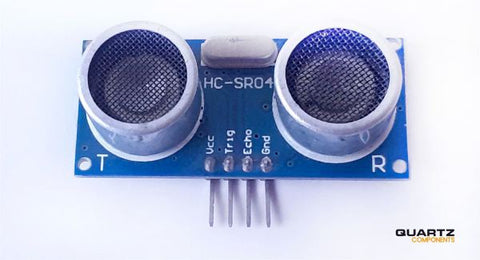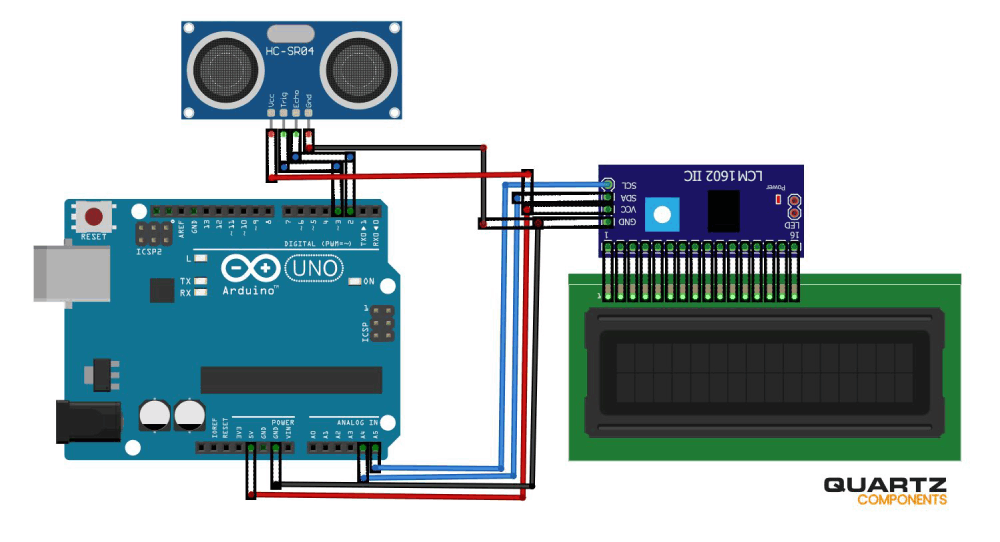# Distance Measurement using Ultrasonic Sensor and Arduino

0 comments / Posted on by Quartz Components

Distance measurement of an object through the air is used in a large number of applications such as obstacle avoider robot control, vehicle control, blind stick, medical application, etc. The ultrasonic sensor is one of the cheapest and easily available option to measure distance for similar requirements. This sensor can be easily interfaced with Arduino or NodeMCU or any other microcontroller and requires only 8 to 10 line code to measure the distance through air.

So here in this DIY project, we are using the Ultrasonic sensor and Arduino Uno to build a distance measurement system. A 16x2 LCD module is also used to display the distance. So let's get started and see the list of required materials for the project.

## Components Required for Arduino Distance Measurement Project## How HC-SR04 Ultrasonic Sensor works

An ultrasonic sensor is a device that is generally used to measures the distance to an object and object detection using ultrasonic sound waves. The sensor head transmits an ultrasonic wave and receives the wave reflected from the object. Ultrasonic Sensors measure the distance to the object by calculating the time between the transmission and reception.

Distance= (Time x Speed of Sound in Air (340 m/s))/2## Circuit Diagram

The circuit diagram for Ultrasonic distance measurement using Arduino is given below.Trig and Echo pins of the ultrasonic sensor are connected to digital pin 3 & 2 of Arduino. VCC pin of the ultrasonic sensor is connected to the 5v pin of Arduino while the GND pin is connected to the GND of Arduino. SDA & SCL pin of the I2C module is connected to the A4 & A5 pin of Arduino while VCC and GND pins are connected to the 5V & GND pin of Arduino.

## Code Explanation

Complete code with a working video for this Arduino and ultrasonic sensor based distance measurement project is given at the end of the document. Here we are briefly explaining the code.

Start the code by including the library files for LCD and Wire. These library files will be used for I2C communication between Arduino and LCD module.

#include <Wire.h>

#include <LiquidCrystal_I2C.h>

LiquidCrystal_I2C lcd(0x27, 16, 2);

Then define the pins where you connected the Trig and Echo pins of the ultrasonic sensor.

const int trigPin = 3;

const int echoPin = 2;

After that, define two variables for duration and distance.

long duration;

int distance;

Inside the void setup function, define the Trig pin as an output pin and Echo pin as an input.

pinMode(trigPin, OUTPUT);

pinMode(echoPin, INPUT);

Now inside the void loop function, calculate the time between triggered and received signal. This time will be used to calculate the distance.

duration = pulseIn(echoPin, HIGH);

distance = duration * 0.0340 / 2;

## Testing the Project

After connecting all the components connect the Arduino to the laptop and upload the program. Now to check whether your project working correctly or not, put an object at different distances and compare the calculated distance with actual distance.

## Complete Code for Arduino Distance Measurement

#include <Wire.h>
#include <LiquidCrystal_I2C.h>
LiquidCrystal_I2C lcd(0x27, 16, 2);
const int trigPin = 3;
const int echoPin = 2;
long duration;
int distance;

void setup() {

lcd.begin(); // Initializes the interface to the LCD display
lcd.backlight();
pinMode(trigPin, OUTPUT);
pinMode(echoPin, INPUT);
Serial.begin(9600);

}

void loop() {
lcd.clear();
digitalWrite(trigPin, LOW);
delayMicroseconds(2);
digitalWrite(trigPin, HIGH);
delayMicroseconds(10);
digitalWrite(trigPin, LOW);
duration = pulseIn(echoPin, HIGH);
distance = duration * 0.0340 / 2;
Serial.println("Distance");
Serial.println(distance);
lcd.setCursor(0, 0);
lcd.print("Distance: ");
lcd.print(distance);
lcd.print("cm");
delay(1000);
}

## Video

Distance measurement of an object through the air is used in a large number of applications such as obstacle avoider robot control, vehicle control, blind stick, medical application, etc. The ultrasonic sensor is one of the cheapest and easily available option to measure distance for similar requirements. This sensor can be easily interfaced with Arduino or NodeMCU or any other microcontroller and requires only 8 to 10 line code to measure the distance through air.

So here in this DIY project, we are using the Ultrasonic sensor and Arduino Uno to build a distance measurement system. A 16x2 LCD module is also used to display the distance. So let's get started and see the list of required materials for the project.

## Components Required for Arduino Distance Measurement Project## How HC-SR04 Ultrasonic Sensor works

An ultrasonic sensor is a device that is generally used to measures the distance to an object and object detection using ultrasonic sound waves. The sensor head transmits an ultrasonic wave and receives the wave reflected from the object. Ultrasonic Sensors measure the distance to the object by calculating the time between the transmission and reception.

Distance= (Time x Speed of Sound in Air (340 m/s))/2## Circuit Diagram

The circuit diagram for Ultrasonic distance measurement using Arduino is given below.Trig and Echo pins of the ultrasonic sensor are connected to digital pin 3 & 2 of Arduino. VCC pin of the ultrasonic sensor is connected to the 5v pin of Arduino while the GND pin is connected to the GND of Arduino. SDA & SCL pin of the I2C module is connected to the A4 & A5 pin of Arduino while VCC and GND pins are connected to the 5V & GND pin of Arduino.

## Code Explanation

Complete code with a working video for this Arduino and ultrasonic sensor based distance measurement project is given at the end of the document. Here we are briefly explaining the code.

Start the code by including the library files for LCD and Wire. These library files will be used for I2C communication between Arduino and LCD module.

#include <Wire.h>

#include <LiquidCrystal_I2C.h>

LiquidCrystal_I2C lcd(0x27, 16, 2);

Then define the pins where you connected the Trig and Echo pins of the ultrasonic sensor.

const int trigPin = 3;

const int echoPin = 2;

After that, define two variables for duration and distance.

long duration;

int distance;

Inside the void setup function, define the Trig pin as an output pin and Echo pin as an input.

pinMode(trigPin, OUTPUT);

pinMode(echoPin, INPUT);

Now inside the void loop function, calculate the time between triggered and received signal. This time will be used to calculate the distance.

duration = pulseIn(echoPin, HIGH);

distance = duration * 0.0340 / 2;

## Testing the Project

After connecting all the components connect the Arduino to the laptop and upload the program. Now to check whether your project working correctly or not, put an object at different distances and compare the calculated distance with actual distance.

## Complete Code for Arduino Distance Measurement

#include <Wire.h>
#include <LiquidCrystal_I2C.h>
LiquidCrystal_I2C lcd(0x27, 16, 2);
const int trigPin = 3;
const int echoPin = 2;
long duration;
int distance;

void setup() {

lcd.begin(); // Initializes the interface to the LCD display
lcd.backlight();
pinMode(trigPin, OUTPUT);
pinMode(echoPin, INPUT);
Serial.begin(9600);

}

void loop() {
lcd.clear();
digitalWrite(trigPin, LOW);
delayMicroseconds(2);
digitalWrite(trigPin, HIGH);
delayMicroseconds(10);
digitalWrite(trigPin, LOW);
duration = pulseIn(echoPin, HIGH);
distance = duration * 0.0340 / 2;
Serial.println("Distance");
Serial.println(distance);
lcd.setCursor(0, 0);
lcd.print("Distance: ");
lcd.print(distance);
lcd.print("cm");
delay(1000);
}